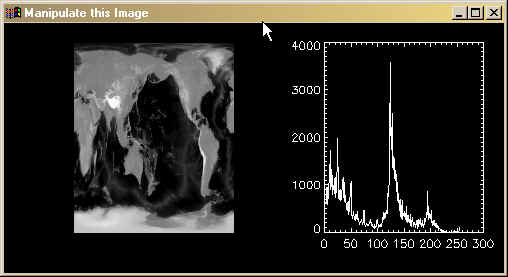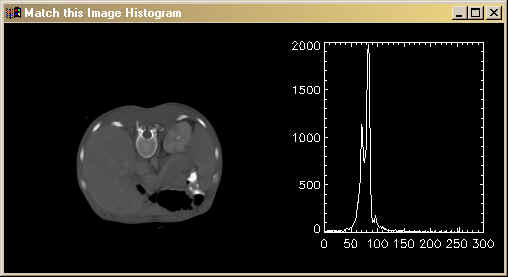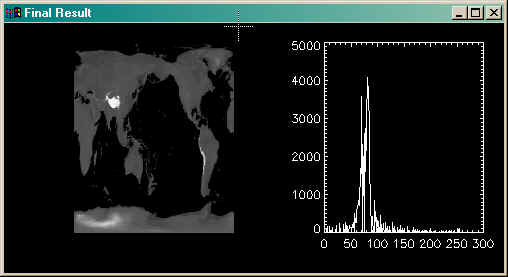Histogram Matching

QUESTION: Is there a function in IDL to perform histogram matching?ANSWER: No, there is no built-in function to do perform histogram matching. But it is not difficult to create such a function in IDL. Here is how to do it. I've used the book Digital Image Processing, Second Edition by Gonzales and Woods as a template for the HistoMatch program that results from this analysis.

Histogram matching is similar in concept to histogram equalization, in which the pixel values of an image are manipulated in such a way as to distribute the pixel values in an approximately even distribution over the dynamic color range of the image. The difference is that in histogram matching we don't use an even distribution, but a distribution that is supplied to us from another image or perhaps from a subset of the same image. In other words, we want to manipulate the pixel distribution of one image to mirror the pixel distribution of another image. The pixel distribution of an image is, of course, its histogram.

To begin, let's open an image we would like to manipulate. Type these commands.

filename = Filepath('worldelv.dat', Subdir=['examples', 'data'])
OpenR, lun, filename, /Get_Lun
image = BytArr(360, 360)
Free_Lun, lun

Display it next to its histogram by typing these commands. You see the result below the text.

Window, 1, XSize=500, YSize=250, Title='Manipulate this Image', XPos=100, YPos=360
!P.Multi=[0,2,1]
TVImage, image
Plot, Histogram(image), Max_Value=5000
!P.Multi=0Next, open an image whose histogram or pixel distribution we want to match. Type these commands.

filename = Filepath('ctscan.dat', Subdir=['examples', 'data'])
OpenR, lun, filename, /Get_Lun
image_to_match = BytArr(256, 256)
Free_Lun, lun

Display it next to its histogram by typing these commands. You see the result below the text.

Window, 0, XSize=500, YSize=250, Title='Match this Image Histogram', XPos=100, YPos=100
!P.Multi=[0,2,1]
TVImage, image_to_match
Plot, Histogram(image_to_match), Max_Value=5000
!P.Multi=0The Histogram Matching Algorithm

Now we are ready to develop the histogram matching algorithm. The first thing to do is calcualte the histograms of both images. Type this code.

match_histogram = Histogram(Byte(histogram_to_match), Min=0, Max=255, Binsize=1)
h = Histogram(Byte(image), Min=0, Max=255,Binsize=1)

In histogram matching we are trying to manipulate the pixel values of one image into the same pixel distribution as another image. The method we are going to use requires that both images have the same number of pixels. This is not the case here, since our images are different sizes (one is 256-by-256 and the other is 360-by-360). Therefore, we need to correct the match_histogram to have the same number of pixels. Type these commands.

totalPixels = Float(N_Elements(image))
totalHistogramPixels = Float(Total(match_histogram))

IF totalPixels NE totalHistogramPixels THEN \$
factor = totalPixels / totalHistogramPixels ELSE \$
factor = 1.0

match_histogram = match_histogram * factor

The next step is to create a vector s representing the transformation function of the image we wish to manipulate. We use its histogram to construct the vector, like this.

s = FltArr(256)
FOR k=0,255 DO BEGIN
s[k] = Total(h(0:k) / totalPixels)
ENDFOR

We do the same thing for the image whose histogram we wish to match.

v = FltArr(256)
FOR q=0,255 DO BEGIN
v[q] = Total(match_histogram(0:q) / Total(match_histogram))
ENDFOR

Now we construct a distribution function z from the vectors s and v by simply adding up pixels in s until we find approximately the same number of pixels as are in v. Note that the process of histogram matching, like histogram equalization, is only approximate. Type this code.

z = BytArr(256)
FOR j=0,255 DO BEGIN
i = Where(v LT s[j], count)
IF count GT 0 THEN z[j] = (Reverse(i)) ELSE z[j]=0
ENDFOR

We create the histogram matched image by simply looking up the image values in the new distribution function. Type this code.

matchedImage = z[Byte(image)]

Here is the new histogram matched image. Notice the tonal values are similar to the image we are trying to match and that its histogram closely follows the shape of the histogram we are trying to match.

Window, 2, XSize=500, YSize=250, Title='Final Result', XPos=100, YPos=630
!P.Multi=[0,2,1]
TVImage, matchedImage
Plot, Histogram(matchedImage ), Max_Value=5000
!P.Multi=0You can perform this kind of histogram matching with the HistoMatch program.Web Coyote's Guide to IDL Programming